# Vorticity facts for kids

Kids Encyclopedia Facts

Vorticity is a mathematical concept used in fluid dynamics. It can be related to the amount of "circulation" or "rotation" (or more strictly, the local angular rate of rotation) in a fluid.

The average vorticity in a small region of fluid flow is equal to the circulation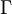$\Gamma$ around the boundary of the small region, divided by the area A of the small region.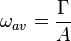$\omega_{av} = \frac {\Gamma}{A}$

Notionally, the vorticity at a point in a fluid is the limit as the area of the small region of fluid approaches zero at the point: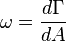$\omega = \frac {d \Gamma}{dA}$

Mathematically, the vorticity at a point is a vector and is defined as the curl of the velocity: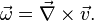$\vec \omega = \vec \nabla \times \vec v .$

One of the base assumptions of the potential flow assumption is that the vorticity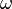$\omega$ is zero almost everywhere, except in a boundary layer or a stream-surface immediately bounding a boundary layer.

Because a vortex is a region of concentrated vorticity, the non-zero vorticity in these specific regions can be modelled with vortices.

• Batchelor, G. K., (1967, reprinted 2000) An Introduction to Fluid Dynamics, Cambridge Univ. Press
• Ohkitani, K., "Elementary Account Of Vorticity And Related Equations". Cambridge University Press. January 30, 2005.
• Chorin, Alexandre J., "Vorticity and Turbulence". Applied Mathematical Sciences, Vol 103, Springer-Verlag. March 1, 1994.
• Majda, Andrew J., Andrea L. Bertozzi, "Vorticity and Incompressible Flow". Cambridge University Press; 2002.
• Tritton, D. J., "Physical Fluid Dynamics". Van Nostrand Reinhold, New York. 1977.
• Arfken, G., "Mathematical Methods for Physicists", 3rd ed. Academic Press, Orlando, FL. 1985.Vorticity Facts for Kids. Kiddle Encyclopedia.